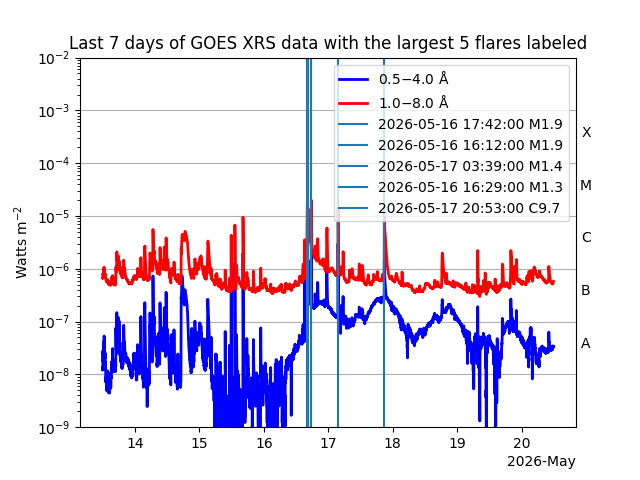# Creating a TimeSeries from GOES-XRS near real time data#

This example will demonstrate how to download and load GOES XRS Near Real Time (NRT) data into a sunpy.timeseries.TimeSeries.

import matplotlib.pyplot as plt
import pandas as pd

from astropy import units as u

from sunpy import timeseries as ts
from sunpy.time import parse_time


We will start by getting reading the GOES-XRS JSON file using pandas.read_json. This allows us to download the file and load it straight into a pandas.DataFrame. This file updates every minute and contains only the last 7 days worth of data.

goes_json_data = pd.read_json("https://services.swpc.noaa.gov/json/goes/primary/xrays-7-day.json")


XRS collects data in two energy channels, “0.05-0.4nm” and “0.1-0.8nm”. We separate these “short” and “long” wavelength readings into two arrays.

# This will get us the short wavelength data.
goes_short = goes_json_data[goes_json_data["energy"] == "0.05-0.4nm"]
# This will get us the long wavelength data.
goes_long = goes_json_data[goes_json_data["energy"] == "0.1-0.8nm"]


sunpy.timeseries.TimeSeries requires a datetime index which we can get directly and transform into astropy.time.Time.

time_array = parse_time(goes_short["time_tag"])


sunpy.timeseries.TimeSeries requires that there are units for data variables. To do this, we will create a dictionary that will map the names of the two columns, “xrsa” and “xrsb” (the channel names for GOES XRS), to their corresponding physical flux units, u.W/u.m**2.

units = dict([("xrsa", u.W/u.m**2), ("xrsb", u.W/u.m**2)])


We need to create a metadata dictionary for the data. Typically, sunpy.timeseries.TimeSeries reads the metadata directly from the file. However, here we need to define our own metadata and we will keep it fairly simple.

meta = dict({"instrument": "GOES X-ray sensor", "measurements": "primary", "type": "quicklook"})


The final pre-step is create a new pandas.DataFrame which we can pass to sunpy.timeseries.TimeSeries as the data input.

goes_data = pd.DataFrame({"xrsa": goes_short["flux"].values, "xrsb": goes_long["flux"].values}, index=time_array.datetime)


Now we will create a sunpy.timeseries.TimeSeries by passing in the data, the metadata and the units.

goes_ts = ts.TimeSeries(goes_data, meta, units, source="xrs")


Finally, we can plot the timeseries.

fig, ax = plt.subplots()
goes_ts.plot(axes=ax)

plt.show()Total running time of the script: (0 minutes 0.814 seconds)

Gallery generated by Sphinx-Gallery